# 时间复杂度 7月1日 【今日算法】-问答-阿里云开发者社区-阿里云

## 时间复杂度 7月1日 【今日算法】游客ih62co2qqq5ww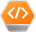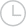2020-07-02 23:54:511354

### 什么是大O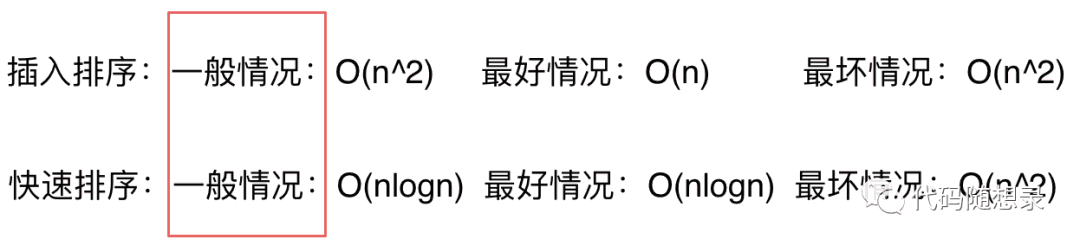### 如何描述时间复杂度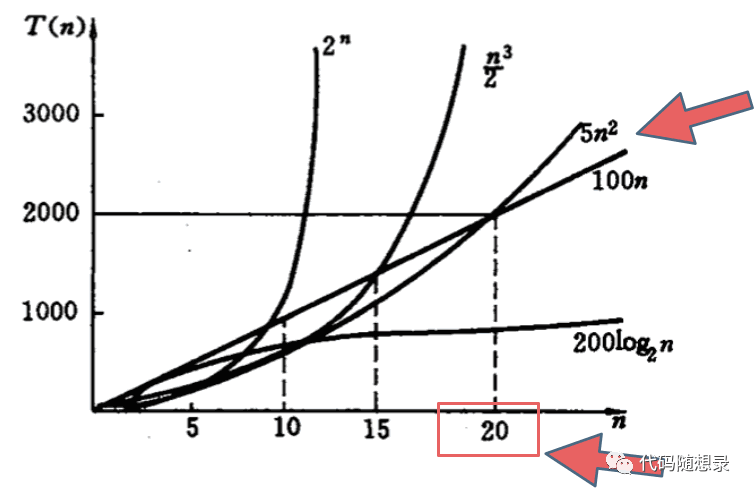``````O(1)常数阶 < O(logn)对数阶 < O(n)线性阶 < O(n^2)平方阶 < O(n^3)(立方阶) < O(2^n) (指数阶)
``````

### 你所不知道的O(logn)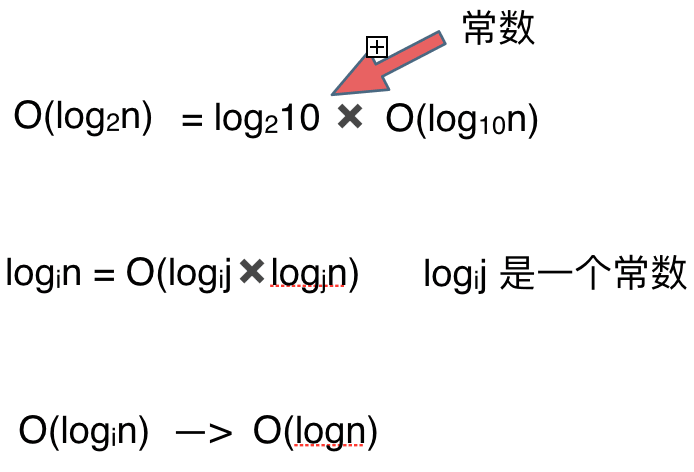### 如果时间复杂度是一个复杂的表达式，我们如何简化

``````O(2*n^2 + 10*n + 1000)
``````

``````O(2*n^2 + 10*n)
``````

``````O(n^2 + n)
``````

``````O(n^2)
``````

``````O(n^2)
``````

``````O(2*n^2 + 10*n + 1000) < O(3*n^2)
``````

### 举例说明时间复杂度要怎么算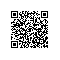取消 提交回答使用钉钉扫一扫加入圈子
+ 订阅